Mobile QR Code1. (Department of Electrical and Electronic Engineering, Hanyang University)
2. (Division of Electrical Engineering, Hanyang University)

Gate-all-around, nanosheet, straineffectiveness, sub-7 nm CMOS

## I. INTRODUCTION

Device density has improved through relentless device scaling and innovative advances in material, process, and device structure. FinFET devices have good electrostatic control over the channel to allow enough drive at limited gate overdrive and off-state current. However, further scaling requires tighter fin pitch and higher fins, that will eventually be limited by increased parasitic elements. Gate-all-around (GAA) field-effect transistors (FETs) that covers all four sides of the channel is advantageous due to its ultimate electrostatic integrity and reasonable compatibility with the FinFET process. Therefore, GAA FETs are attracting attention as a strong candidate to succeed FinFETs in sub-7nm CMOS technology.

Both FinFETs and GAA FETs can increase the drive current for a given footprint by expanding in the vertical direction. Recent experimental results show promising demonstrations of short gate length (LG < 15 nm), tight pitch GAA FETs with multiple stacked channel layers [1,3]. However, this is accompanied at a cost of increased parasitic capacitance that will eventually limit the circuit performance. Hence, it is becoming difficult to improve the drive current of Si(Ge) channels without sacrificing circuit speed. Strain has been a performance enhancer for many technology nodes to boost the carrier mobility. Strain is incorporated in a GAA device by multi-layered epitaxy of alternating Si/SiGe layers, source/drain regrowth epitaxy, and stressor deposition. Therefore, a comprehensive study on the strain effectiveness of GAA devices is necessary. Few simulation studies have been performed on strained GAA devices in prior art. Particularly, there are not much studies of strain effectiveness that depend on surface orientation, channel direction, and cross-section of GAA devices.

In this paper, we investigate the strain effect on GAA FETs with various channel directions and cross-sections under uniaxial compressive and tensile stress. For a rectangular GAA FET that has four channels, the sidewall surface orientation changes with channel direction resulting in different stain-induced current enhancement factors. Furthermore, the improvement of the strained GAA channel depends on the areal ratio of top and bottom surfaces to the sidewalls. Finally, we find the strain requirements for GAA FETs with various cross-sectional widths to meet the drive current specification for a given footprint presented in the International Roadmap for Devices and Systems (IRDS) for 7 nm technology.

## II. SIMULATION METHODOLOGY

### 1. Device Structure

Fig. 1 shows three device configurations used for simulation using Synopsys SentaurusTM Device. The crystal orientation of the top/bottom surface is fixed at (001) and the channel directions < 100 > and < 110 > are used.

Fig. 1. Illustration of the three configurations of GAA FETs used in this work. Surface orientation and channel directions are denoted. Case 3 differs by having a tall cross-sectional shape. EOT = 0.8 nm is used, and ohmic contacts are used for the source/drain electrodes.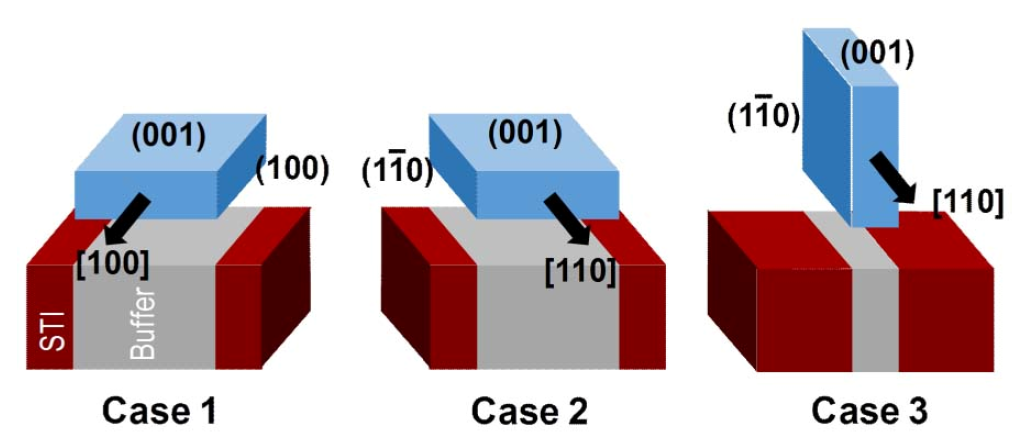The sidewall surface orientation depends on the channel direction. Cases 2 and 3 have the same surface orientations but have different top/bottom-to-sidewall areal ratios. Case 2 has a wide cross-sectional shape with a width of 30 nm and a height of 10 nm, while Case 3 has a tall channel with width and height of 10 nm and 30 nm, respectively. The dominant channel surfaces are the top/bottom for Cases 1 and 2, while it is the sidewalls for Case 3. Table 1 shows the combinations of surface orientation, channel direction, and cross-sectional shapes.

Table 1. Device configurations used for simulations

 Channel Direction Sidewall Orientation Cross Section Case 1  (100) Wide Case 2  (110) Wide Case 3  (110) Tall

### 2. Physical Models

For stress conditions, uniaxial tensile and compressive strain were applied up to ±2 %. The direction of the applied stress is parallel to the channel direction. For the strain models, a multi-valley analytical k ×p band structure and deformation potentials are used. Degeneracy of the bands are lifted by uniaxial strain, and strain-dependent effective mass and density-of-states are captured through occupancy-weighted band minima parameters. Quantum effects are also considered to capture the quantization of energy levels due to spatial confinement and selfconsistent confined carrier distributions.

### 3. Model Verification

Transport model parameters, i.e. fitting parameters for phonon and surface roughness scattering, for different surface orientations were calibrated to match the electron mobility of experimental results[9,10]. Then, we compare the mobility enhancement factor ($\mu_{strained}$/$\mu_{unstrained}$) due to uniaxial tensile stress with that in the literature. Table 2 juxtaposes the results from Ref.  and that of this work. The stress-dependent electron mobility values are in good agreement for three different surface orientation/direction combinations at two different stress values.

Table 2. Comparison of the strain-induced mobility enhancement factors ($\mu_{strained}$/$\mu_{unstrained}$)

 Crystal Orientation Channel Direction Tensile 0.5 GPa Tensile 1.0 GPa Ref.  This Work Ref.  This Work (100) < 100 > 1.35 1.36 1.45 1.46 (100) < 110 > 1.23 1.22 1.55 1.52 (110) < 110 > 1.25 1.22 1.55 1.52

## III. RESULTS AND DISCUSSION

### 1. Strain Effectiveness of GAA FETs

Due to strain, two main differences in current-voltage ($I_{D^{-}} V_{G S}$) characteristics are manifested: threshold voltage shift ($\Delta V_{t h}$) and change in drive current. Both have a significant implications to the system, where the $V_{t h}$ affects the off-state power consumption while the drive current directly affects the device and circuit performance.

Fig. 2 shows the $\Delta V_{t h}$ of GAA FETs as a function of strain. For both compressive and tensile strain conditions, the $V_{t h}$ shifts in the negative direction. Uniaxial strain lifts the energy degeneracy of the band minima valleys. The increase (or decrease) of the valley energies depend on deformation potentials, crystal orientation, channel direction, tensile/compressive, and stress amount. As some valley energies are increased, others are decreased which generally leads to a decrease in the band gap due to the lowered valley energies. Previous analytical work show the strain-dependent $\Delta V_{t h}$ can be expressed by the following expression[12,13]:

Fig. 2. Threshold voltage shift due to compressive and tensile strain obtained (a) via simulation (this work), (b) analytical expression given by Eq. (1).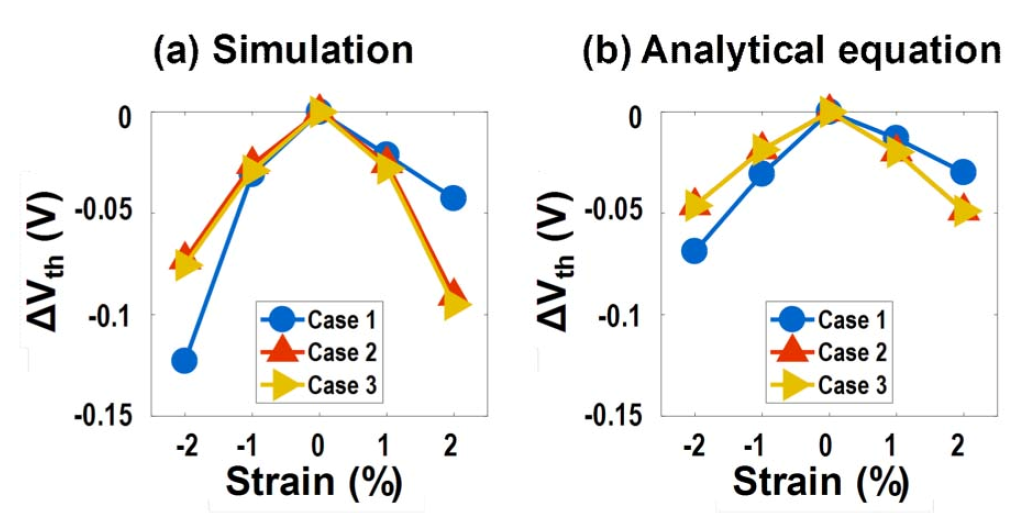##### (1)
${t}_{s}={t}_{sk}\left(forehead\right)×0.07+{t}_{sk}\left(forearm\right)×0.14\phantom{\rule{0ex}{0ex}}+{t}_{sk}\left(backofthehand\right)×0.05+{t}_{sk}\left(abdomen\right)×0.35\phantom{\rule{0ex}{0ex}}+{t}_{sk}\left(upperthighs\right)×0.19+{t}_{sk}\left(lowerthighs\right)×0.13\phantom{\rule{0ex}{0ex}}+{t}_{sk}\left(foot\right)×0.07$

where m is body effect coefficient, $N_{v}$ is the effective density of states in the valence band, and e is the strain amount. Eq. (1) suggests that although present, the $\Delta V_{t h}$ due to strain-induced change in density-of-states or mobility ($\mu_{e}$) are minimal. Fig. 2 shows that the $\Delta V_{t h}$ result obtained by using Eq. (1) agree with that from the simulation for various strain values.

Fig. 3 shows the variation in drive current ($I_{O N}$) as a function of the applied strain. Since electrons redistribute to increase the occupancy of lower energy valleys, strain conditions can be optimized to reduce the valleyaveraged effective mass. Furthermore, due to the energy split, inter-valley scattering is reduced. Fig. 3(a) includes the effect of both $\Delta V_{t h}$ and $\Delta \mu_{e}$, while Fig. 3(b) excludes $\Delta V_{t h}$ effect and solely shows the transport aspect as the $I_{off}$ is fixed for all devices. For Case 1, the average effective mass saturates to the transverse effective mass ($m_{t}$ = 0.19 $m_{0}$) as the occupancy of ellipsoidal conduction band valleys with $m_{t}$ dominates. Hence, the mobility saturates at strain values larger than 1%. On the other hand, for Cases 2 and 3, the total effective mass in the transport direction continues to increase due to the band warping via shear strain and reduction of $m_{t}$ in the direction parallel to the applied strain. As a result, the mobility of GAA devices of Case 2 and 3 exceed that of Case 1 at high strain values, and hence exhibit higher drive current. As for the differences between Cases 2 and 3, the smaller top/bottom-to-sidewall areal ratio provides a slight advantage for Case 3 devices in the tensile strain range.

Fig. 3. Drive current of devices with different configurations, with from compressive -2% to tensile 2% uniaxial strain. All drive currents are normalized to the unstrained GAA device of Case 1. Two scenarios are shown (a) $I_{off}$ is fixed only for the unstrained cases, so the strain-dependent $\Delta V_{t h}$ is accounted in the current values, (b) $I_{off}$ is fixed at 100 nA/μm for all devices, to ignore $\Delta V_{t h}$ effects and show only the influence of the straindependent mobility.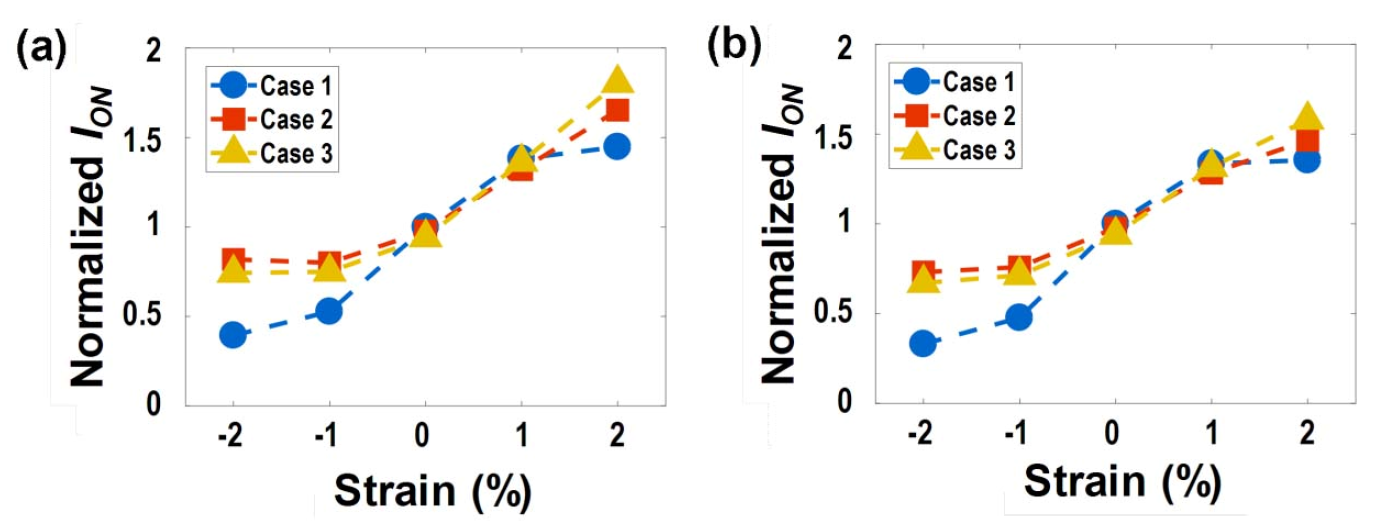### 2. Impact of GAA Device Width

Previous studies have shown wide nanosheet structures that fully utilize the device footprint and maximize the effective width $W_{eff}$[1,2]. In this section, we highlight the importance of the nanosheet width for a given device footprint. As in the case of FinFETs, multiple GAA FETs can be placed provided that the specified device footprint is sufficiently wide. Fig. 4 shows a cross-sectional diagram of multiple devices, and the notations used for this work. Only the width of the device footprint (FW) and the cross-sectional width of the nanosheet (WNS) are varied. The device-to-device spacing (S) is expressed as S = [FW – (N × WNS)] / (N - 1), where N is the number of GAA FETs, and is fixed at 10 nm[16,17]. Hence, NS of the GAA FET determines how many devices can fit in the FW. To investigate the quantized nature of the drive current ($I_{ON}$) of GAA FETs, we compare the $I_{ON}$ for a given FW of uniaxially-tensilestrained GAA FETs with various different WNS and FW. WNS of 10, 20, and 40 nm are used based on recent experimental studies. Device dimensions and conditions are taken from 7 nm technology of the IRDS: $L_{G}$ = 14 nm, $V_{DD}$ = 0.7 V, $I_{OFF}$ = 100 nA/μm. To compare the intrinsic device performance, external series resistance effects are not included.

Fig. 4. Schematic diagram of multiple GAA FETs within a given device footprint (FW). Cross-sectional width (WNS) and height (HNS), spacing (S) are denoted.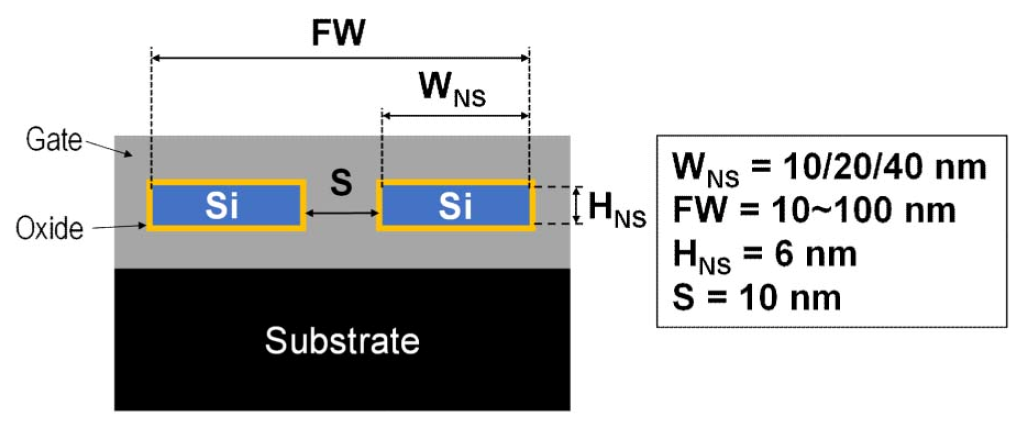Fig. 5 shows the $I_{ON}$ for GAA devices with a channel direction of < 100 > and < 110 > , under tensile strain values of 0, 1, and 2 %. Single-stack GAA FETs are used to focus on the effect of strain and choice of WNS, since gains in drive current of multi-layered GAA do not directly translate to increase in circuit speed due to worse parasitic capacitance. The solid line indicates the NMOS drive current requirement, based on the IRDS. Fig. 5 shows that depending on the WNS and FW, devices may or may not meet the $I_{ON}$ requirement. Choice of device width is crucial in maximizing the effective width of the GAA device for a given footprint. In most cases, $I_{ON}$ is higher for larger WNS devices, but in terms of layout design flexibility smaller WNS devices are preferable. Strain boosts the $I_{ON}$ of a single-stack GAA device without suffering from a trade-off with increased parasitic capacitance. The < 110 > channel direction has a larger current than the < 100 > direction at higher strain values ($\varepsilon$ = 2%), since the mobility saturates for the < 100 > direction devices.

Fig. 5. GAA NMOS drive current $I_{ON}$ against the device footprint width (FW). Device conditions are $L_{G}$ = 14 nm, $V_{DD}$ = 0.7 V, $I_{OFF}$ = 100 nA/μm (a)-(c) Surface orientation/channel direction of (001) / < 100 > , (d)-(f) (001)/ < 110 > . Solid line is the specification from the IRDS. FW = 10 nm is denoted by a dotted line.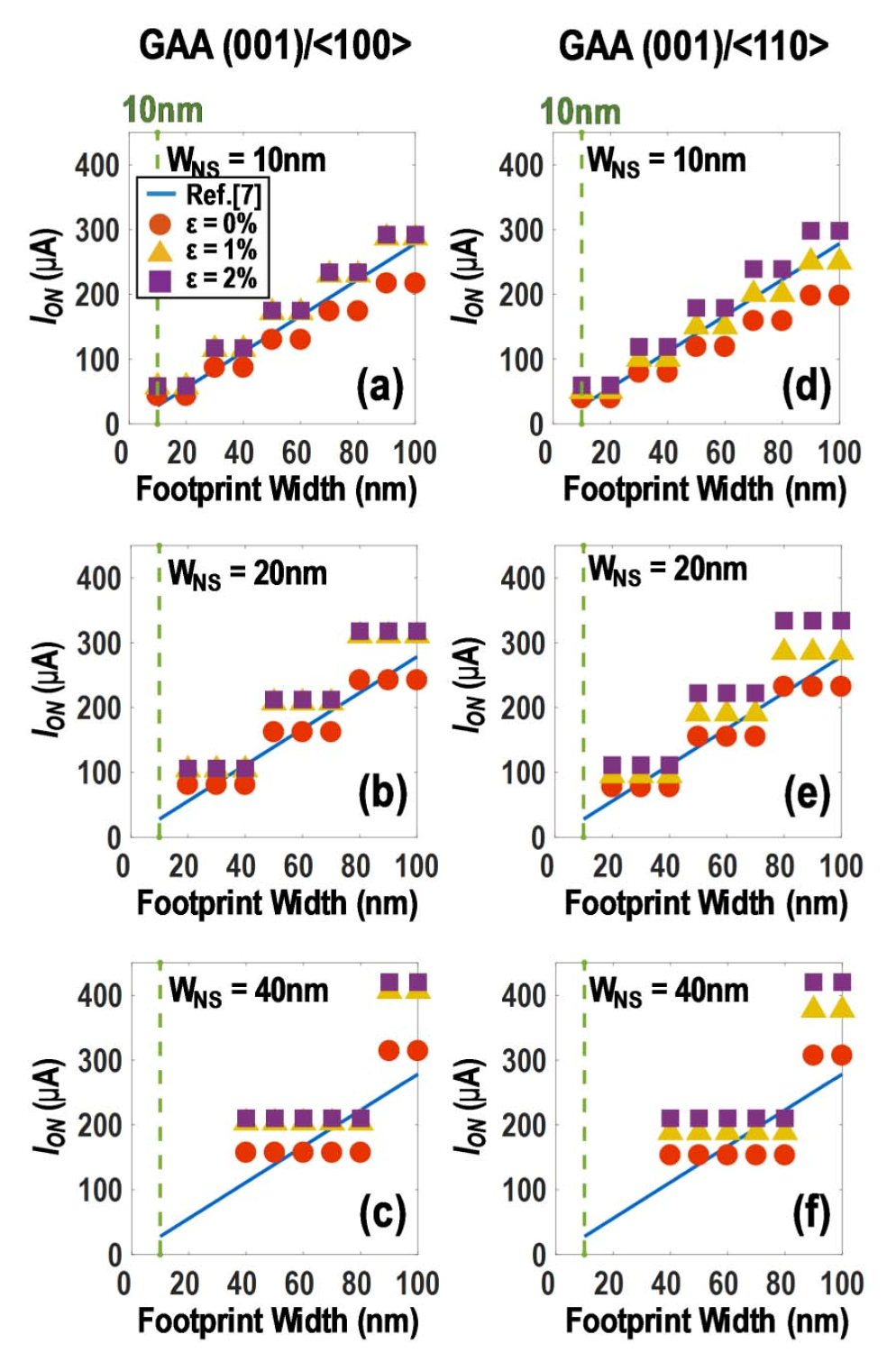## IV. CONCLUSION

This work studied the strain effectiveness of GAA FETs with various surface orientations and cross-sections. A negative Vth shift is caused by a decrease in bandgap via either compressive or tensile strain. Uniaxial tensile strain shows the highest drive current for (001)/ < 100 > configuration up to 1% strain, while the (001)/ < 110 > configuration has the drive current for higher strain values. For GAA NMOS to satisfy the drive current requirements, choice of device width is crucial to fully utilize the entire device footprint. For a given layout, effective strain incorporation enhances the drive current of a single-stack GAA FET to satisfy drive current requirements without introducing limitations of increased parasitic capacitance.

### ACKNOWLEDGMENTS

This work was supported by the research fund of Hanyang University (HY-2016-N, HY-2017-N). The EDA tool was supported by the IC Design Education Center (IDEC), Korea.

### REFERENCES

1
Loubet N., et al , June 2017, Stacked nanosheet gate-allaround transistor to enable scaling beyond finFET, in Proc. Symp. VLSI Technol., pp. 230-2312
Mertens H., et al , Dec. 2017, Vertically stacked gate-allaround Si nanowire transistors : key process optimizations and ring oscillator demonstration, in IEDM Tech. Dig., pp. 828-8313
Zhang J., et al , Dec. 2017, High-k Metal Gate Fundamental Learning and Multi-VT Options for Stacked Nanosheet Gate-All-Around Transistor, in IEDM Tech. Dig., pp. 537-5404
Barraud S., et al , June 2017, Vertically Stacked-NanoWires MOSFETs in a Replacement Metal Gate Process with Inner Spacer and SiGe Source/Drain, in IEDM Tech. Dig., pp. 464-6475
Elmessary M. A., et al , Sep. 2017, Study of Strained Effects in Nanoscale GAA Nanowire FETs Using 3D Monte Carlo Simulations, in ESSDERC, pp. 184-1876
Al-Ameri T., et al , Sep. 2016, Impact of strain on the performance of Si nanowires transistors at the scaling limit: a 3D Monte Carlo / 2D Poisson Schrodinger simulation study, in SISPAD, pp. 213-2167
International roadmap for devices and systems, 2016 edition, https://irds.ieee.org/reports8
Sentaurus Device, version M-2016.12, Synopsys, Inc., Mountain View, CA, USA9
Mujtaba S. A., et al , 1994, Semi-empirical local NMOS mobility model for 2-D device simulation incorporating screened minority impurity scattering, in Proc. 5th Intl. Workshop on Numerical Modeling of Processes and Devices for Integrated Circuits (NUPAD), pp. 3-610
Mujtaba S. A., et al , 1995, Accurate modeling of Coulombic scattering, and its impact on scaled MOSFETs, in Proc. Symp. VLSI Technol., pp. 99-10011
Tan Y., et al , 2008, Analytical Electron-Mobility Model for Arbitrarily Stressed Silicon, IEEE Trans. Electron Devices, Vol. 55, pp. 1386-139012
Kang T., 2012, Evidence for Silicon Bandgap Narrowing in Uniaxially Strained MOSFETs Subjected to Tensile and Compressive Stress, IEEE Trans. Electron Devices, Vol. 33, pp. 770-77213
Zhang W., et al , 2008, On the Threshold Voltage of Strained-Si-Si1-xGex MOSFETs, IEEE Trans. Electron Devices, Vol. 52, pp. 263-26814
Dhar S., et al , 2005, Electron Mobility Model for Strained-Si Devices, IEEE Trans. Electron Devices, Vol. 52, pp. 527-53315
Uchida K., et al , Dec. 2005, Physical mechanisms of electron mobility enhancement in uniaxial stressed MOSFETs and impact of uniaxial stress engineering in ballistic regime, in IEDM Tech. Dig., pp. 129-13216
Barraud S., et al , Dec. 2017, Performance and Design Considerations for Gate-All-Around Stacked-NanoWires FETs, in IEDM Tech. Dig., pp. 677-68017
Martens H., et al , Dec. 2017, Vertically Stacked Gate-All-Around Si Nanowire Transistors : Key Process Optimizations and Ring Oscillator Demonstration, in IEDM Tech. Dig., pp. 829-831## Author

received the B.S. degree in the Department of Electronic Engineering from Korea Polytechnic Univeristy, Korea, in 2017.

He is currently pursuing the M.S. degree in the Department of Electrical and Electronic Engineering from Hanyang University, Korea.

His interests include high-performance nanoscale devices, oxide thin-film transistors, and synaptic devices.

received the B.S. degree in Electrical Engineering from Korea Advanced Institute of Science and Technology (KAIST), Daejeon, Korea, in 2004, and the M.S. and Ph.D. degrees in Electrical Engineering from Stanford University, Stanford, CA, in 2006 and 2010, respectively.

He joined LG Display, Paju, Korea, in 2010. In 2016, he joined Hanyang University, Ansan, Korea, as an Assistant Professor in the Division of Electrical Engineering.

His research interests include device physics and modeling of low-dimension nanoscale devices, and metal-oxide thinfilm transistor technology for flexible electronics.